## Example Questions

2 Next →

### Example Question #631 : Gre Quantitative Reasoning

Solve for: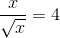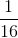Explanation:

To get rid of the radical, multiply top and bottom by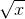.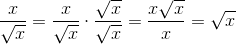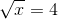Square both sides.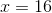### Example Question #52 : Basic Squaring / Square Roots

Simplify: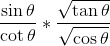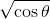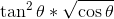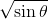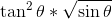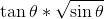Explanation: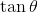is "opposite over adjacent," or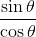. (This is because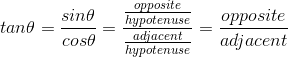.)

Let's substituteinto the equation forand multiply the numerator and the denominator by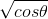.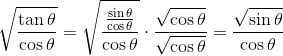Now we can multiply the result by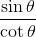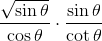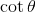is the inverse of, or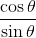: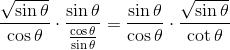We can reduce the resultingto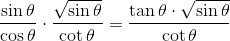By multiplying top and bottom by, we can cancel out thein the numerator: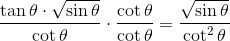The resulting fraction can be simplified: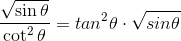### Example Question #11 : How To Divide Square Roots

Simplify.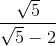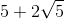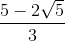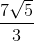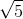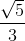Explanation:

To get rid of the radical, we need to multiply by the conjugate. The conjugate uses the opposite sign and multiplying by it will let us rationalize the denominator in this problem. The goal is getting an expression of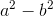in which we are taking the differences of two squares.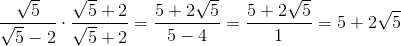2 Next →

### All GRE Math Resources